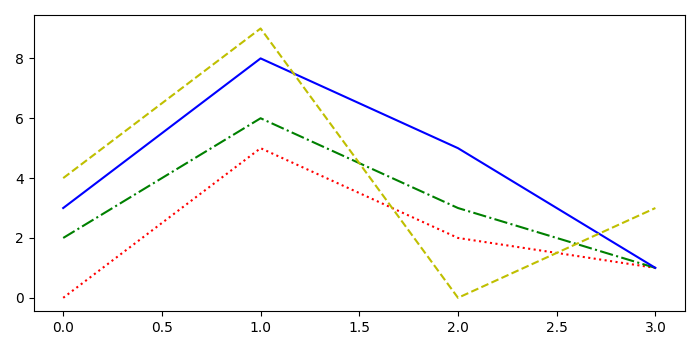# How to cycle through both colours and linestyles on a matplotlib figure?

To cycle through both colors and linestyles on a matplotlib figure, we can take the following steps.

## Steps

• Set the figure size and adjust the padding between and around the subplots.

• Set the current rcParams, withcolors and linestyle.

• Plot the data points using plot() method.

• To display the figure, use Show() method.

## Example

import matplotlib.pyplot as plt
from cycler import cycler

# Set the figure size
plt.rcParams["figure.figsize"] = [7.00, 3.50]
plt.rcParams["figure.autolayout"] = True

# Set the rcParams with color or linestyle
plt.rc('axes', prop_cycle=(cycler('color', ['r', 'g', 'b', 'y']) +
cycler('linestyle', [':', '-.', '-', '--'])))

# Plot the data points
plt.plot([0, 5, 2, 1])
plt.plot([2, 6, 3, 1])
plt.plot([3, 8, 5, 1])
plt.plot([4, 9, 0, 3])

plt.show()

## Output

It will produce the following output −